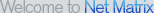# Find Equation Software

Software Search:

Try our new Live Software Search. Just start typing your keywords below and watch the software magically appear!

Enter Keywords:

Math Homework Maker
The Math Homework Maker,is a software which can solve all your math homework. If you are a parent,pupil,student,needing help or just want to check your math homework, Then you must have this software. This software is very easy and friendly to use

Equation Illustrator V
Equation Illustrator V is designed to ease the production of single page handout type documents and web graphics that include math/chemical equations simple vector graphics and pictures.

Functions
Visualizes and studies functions of one variable to find roots, extrema, integral, derivatives, graph. Results can be saved or printed. You can copy the graph to the clipboard. You have one-click control of the graph with zooming, panning, centering

Basic Algebra Shape-Up
Basic Algebra Shape-Up helps students master specific basic algebra skills. Concepts covered include creating formulas; using ratios, proportions, and scale; working with integers, simple and multi-step equations.

Atoms, Symbols and Equations
Unique interactive multimedia Chemistry teaching software that tests students as they learn. Topics include: elements, atoms and molecules, word equations, chemical symbols, Periodic Table, chemical formulas, balancing chemical equations.

3DMath Explorer
3DMath Explorer is a 3D Graph Plotting Software for Math, Science and Engineering. Features: 3D curve ploting in real time, perspective drawing, graph zooming, active graph rotation, fogging effect, cubic draw, unlimited space ploting, four view plot

1D Heat Transfer
The 1D Heat Transfer software is used for to study one-dimensional heat transfer (steady and unsteady states). It can be used for the geometries: wall, Lx = width; long cylinder, Lx = length; sphere, Lx = R/3 and semi-infinite wall.

Infinity
These days, there are many things that require mathematical modeling: from exchange rates prediction to engineering and financial planning. Infinity is the unique math application that brings actual mathematical modeling results! Download FREE trial!

Linear Algebra
Performs computations associated with matrices: Solves systems of linear equations, finds sum, difference, product, determinant, trace, inverse, adjoint, transpose, eigenvalues, eigenvectors, LU factors, QR factors, tests for definiteness, etc.

DataFit
DataFit is a tool for performing curve fitting, statistical analysis & data plotting. Fit equations with up to 100 parameters to data with up to 20 independent variables. Define your own equations or choose from over 600 pre-defined equations.

More Software: 12
Page / 1 / 2